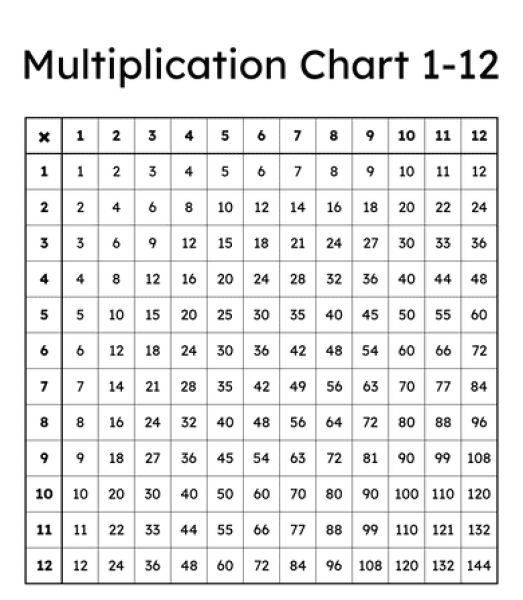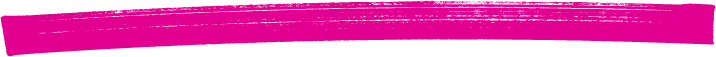# Mastering times tables. A guide for parents.

Last Updated: 17 Nov 23This article provides a guide for parents of young children looking to support them in learning their times tables.

### Mastering Times Tables: A Guide for Parents

Every parent eventually faces the challenge of helping their child learn their times tables. While some children find this task easier than others, all children can benefit from a bit of guidance. Mastering times tables is crucial for a child's math skills, as it paves the way for a more comfortable journey through mathematics in school and adult life.

##### Multiplication Table

Before we look into effective ways to help your child learn times tables, let's look at the multiplication table below. Our primary teachers at Teachers To Your Home suggest three easy ways to help your child remember their times tables.Learning times tables is about repetition and practice. A common mistake is to go through the numbers in order, which can be overwhelming. Instead, start with the easiest tables first. This builds your child's confidence and makes the transition to more challenging tables smoother.

Here's a recommended order for learning times tables:

• The 10s: Begin with the ten times tables, which are the simplest to grasp. Explain that multiplying by 10 involves adding a zero to the number (e.g., 1 x 10 = 10).
• The 5s: The five times tables are relatively straightforward. Encourage your child to memorise these by reciting them rhythmically: "Five, ten, fifteen, twenty..." This can be reinforced through games like counting by fives.
• The 11s: Learning the eleven times tables is relatively easy up to nine. It involves doubling the number (e.g., 2 x 11 = 22). For larger numbers, teach your child a handy trick to multiply by eleven (e.g., 3 x 11 = 33).
• The 2s: When introducing the two times tables, explain the concept of doubling. For instance, 2 x 6 is equivalent to "double six," resulting in 12.
• The 4s: The four times tables can be tackled by doubling the number and then doubling the result. For example, 4 x 5 is 5 + 5 = 10, and then 10 + 10 = 20.
• The 9s: Teach your child a valuable trick to master the nine times tables using their hands. This technique involves recognising patterns based on the position of fingers on each hand.
• The 6s: These tables can be approached by emphasising the concept of multiplication as sequential addition. Utilise objects like coins or pebbles to illustrate the process.
• The 12s: Explore the twelve times tables once your child is comfortable with the earlier tables. Reinforce the idea that multiplication is a form of sequential addition.
• The 8s: As you delve into the eight times tables, continue to emphasise the doubling concept.
• The 7s: The seven times tables can be tricky but are manageable with practice and patience. Encourage your child to visualise the process using practical objects.

Explain how multiplication works. Use objects like Lego, coins, or pebbles to illustrate the concept. For instance, with the 2s, emphasise that you're doubling the number (e.g., 2 x 6 = 6 + 6 = 12). Additionally, multiplication gives the same result forwards and backwards (1 x 2 is the same as 2 x 1).

##### 2. Teach the tricks

• 2 Times Tables: Double the number. For example, 6 x 2 is 6 + 6, which equals 12.
• 4 Times Tables: Double the number, then double the answer. So, 4 x 5 is 5 + 5 = 10, and 10 + 10 = 20, making 4 x 5 equal to 20.
• 9 Times Tables: Use the "palm trick." Assign the left hand for tens and the right hand for ones. For 3 x 9, put down the third finger on your left hand, leaving 2 to the left and 7 to the right. This gives you 27.
• 11 Times Tables: Up to nine, simply double the number (e.g., 2 x 11 = 22). For larger numbers, add the first and second digits, place the answer between them, and carry any tens. For example, 11 x 23 would be 2 + 3 = 5, so the answer is 253

##### 3. Drill, drill, drill, ...

Repetition is critical to mastering times tables. Practice during everyday activities like driving to school, shopping, or bath time. Start with ordered drilling, then mix it up as your child becomes more comfortable. Short drills of 5 to 10 minutes, done 2 to 3 times a day, can accelerate the learning process.

Mastering times tables is a gradual process that demands commitment and patience. Encourage your child to embrace this journey by introducing times tables in a fun and interactive manner. Be actively involved, track their progress, and consider implementing clever tricks to simplify learning. If you find your child struggling with times tables, don't hesitate to seek the assistance of a private tutor. Your dedication and support will undoubtedly contribute to their mathematical success. If you need extra assistance, consider seeking help from a primary teacher or tutor. Good luck on this mathematical journey with your child!

##### We only offer qualified teachersfor home or online tuition

We're better than tutors. We're teachers available and ready to work for you.